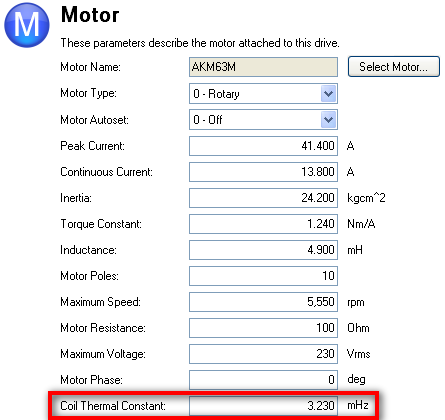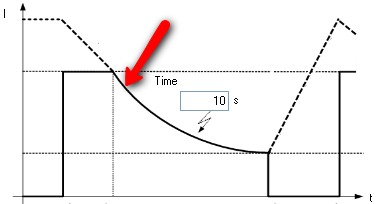Coil Thermal Constant (MOTOR.CTF0)

The Coil Thermal Constant, along with the peak current setting, determines the rate of change of the decrease in motor current during a foldback condition.  When creating a custom motor in Workbench, you will need to know the Coil Thermal Constant.  Since this parameter is not provided for most motors, you can calculate it using the formula below.Corrected formula:

Coil Thermal Constant in mHz = 1000 / (2 * PI * (Thermal Time Constant in sec. / 30.0))

The Customer motor settings in Workbench has the Coil Thermal Constant in mHz.Raviteja Chivukula
Hi Team!

In the above corrected formula, why there is a factor of '30' that's dividing the Time Constant.?

In other words, my question is which expression should be used for analyzing system response. For example, for KBM(S)-35H03-C, the Coil_Thermal_Constant_in_mHz is given as 2.773 mHz. Which of the below two results should be used as time constant for analyzing the first order system response(thermal response)?
- 1000/(2 * Pi * 2.773)
(or)
- 30000/(2 * Pi * 2.773)

Regards
Raviteja Chivukulagby
Hi Raviteja ,

Thanks for finding our mistake.  The issue is that thermal time constants are long enough that it is often convenient to use minutes instead of seconds and the listed formulae incorrectly does the sec to minutes conversion.

As you point out, if the frequency is in mHz, then the time constant in seconds should be

Time constant in sec = 1000/(2*pi*(f in mHz)).

If you then want to convert from seconds to minutes you divide by 60 and get

Time constant in min = 1000/(2*pi*(f in mHz))/60 = 25/(3*pi*(f in mHz))

Hopefully jcoleman02 (posting author) or an admin can update this page soon.

gby
Chief Engineer Servo Technology